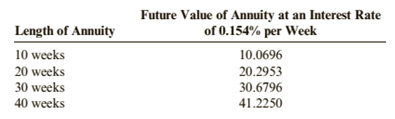Chapter A3, Problem 21E### Cornerstones of Financial Accounti...

4th Edition
Jay Rich + 1 other
ISBN: 9781337690881

#### Solutions

Chapter
Section### Cornerstones of Financial Accounti...

4th Edition
Jay Rich + 1 other
ISBN: 9781337690881
Textbook Problem
1 views

# Devon National Bank pays 8% interest compounded weekly (0.154% per week) on savings accounts. The bank has asked your help in preparing a table to show potential customers the number of dollars that will be available at the end of 10-, 20-, 30-, and 40-week periods during which there are weekly deposits of \$1, S5, \$10, or \$50. The following data are available:Required:Complete a table similar to the one below. (Note: Round answers to two decimal places.)To determine

Introduction:

Compound interest considers the time value of money. Future value of annuity means the value of annual cash flows along with the compound interest on a specific date.

To complete:

The table that shows future value of depositing various amounts for specified period.

Explanation

Given:

 Length of Annuity Future Value of Annuity (where i = 0.1554% per week) 10 weeks 10.0696 20 weeks 20.2953 30 weeks 30.6796 40 weeks 41.2250

Calculations:

Compounded Weekly,

Interest Rate = 0.154%

FV = Future Value

(1a) Annual Cash Flow (f) = \$1 No. of periods = 10

FV = (f)(FV of an annuity, 10 periods, 0.154%)

Using the given ‘FV of an annuity table’,

FV = (\$1)(10.0696)

FV = \$10.07

(1b) Annual Cash Flow (f) = \$1 No. of periods = 20

FV = (f)(FV of an annuity, 20 periods, 0.154%)

Using the given ‘FV of an annuity table’,

FV = (\$1)(20.2953)

FV = \$20.30

(1c) Annual Cash Flow (f) = \$1

No. of periods = 30

FV = (f)(FV of an annuity, 30 periods, 0.154%)

Using the given ‘FV of an annuity table’,

FV = (\$1)(30.6796)

FV = \$30.68

(1d) Annual Cash Flow (f) = \$1

No. of periods = 40

FV = (f)(FV of an annuity, 40 periods, 0.154%)

Using the given ‘FV of an annuity table’,

FV = (\$1)(41.2250)

FV = \$41.23

(2a) Annual Cash Flow (f) = \$5 No. of periods = 10

FV = (f)(FV of an annuity, 10 periods, 0.154%)

Using the given ‘FV of an annuity table’,

FV = (\$5)(10.0696)

FV = \$50.35

(2b) Annual Cash Flow (f) = \$5 No. of periods = 20

FV = (f)(FV of an annuity, 20 periods, 0.154%)

Using the given ‘FV of an annuity table’,

FV = (\$5)(20.2953)

FV = \$101.48

(2c) Annual Cash Flow (f) = \$5

No. of periods = 30

FV = (f)(FV of an annuity, 30 periods, 0.154%)

Using the given ‘FV of an annuity table’,

FV = (\$5)(30.6796)

FV = \$153.398

(2d) Annual Cash Flow (f) = \$5

No...

### Still sussing out bartleby?

Check out a sample textbook solution.

See a sample solution

#### The Solution to Your Study Problems

Bartleby provides explanations to thousands of textbook problems written by our experts, many with advanced degrees!

Get Started